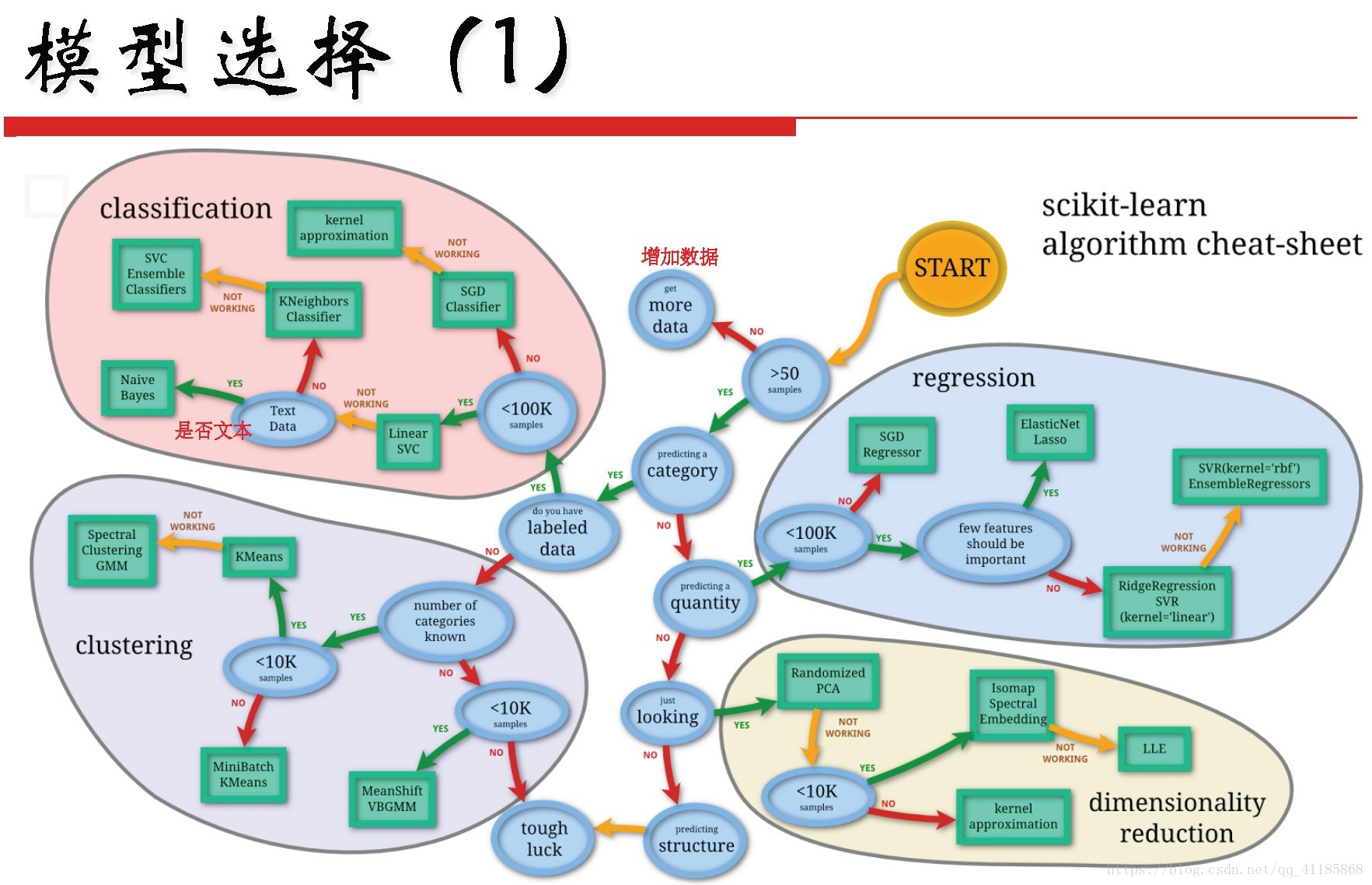+关注继续查看

# 作用

jQuery 元素选择器和属性选择器允许您通过标签名、属性名或内容对 HTML 元素进行选择。

# 元素选择器

jQuery 使用 CSS 选择器来选取 HTML 元素。

$("p") 选取 <p> 元素。$("p.intro") 选取所有 class="intro" 的 <p> 元素。

$("p#demo") 选取所有 id="demo" 的 <p> 元素。 # 属性选择器 jQuery 使用 XPath 表达式来选择带有给定属性的元素。$("[href]") 选取所有带有 href 属性的元素。

$("[href='#']") 选取所有带有 href 值等于 "#" 的元素。$("[href!='#']") 选取所有带有 href 值不等于 "#" 的元素。

$("[href$='.jpg']") 选取所有 href 值以 ".jpg" 结尾的元素。

# CSS 选择器

jQuery CSS 选择器可用于改变 HTML 元素的 CSS 属性。

<span style="font-size:18px;"><span style="font-size:18px;">$("p").css("background-color","red");</span></span> # 更多的选择器实例 语法 描述$(this) 当前 HTML 元素
$("p") 所有 <p> 元素$("p.intro") 所有 class="intro" 的 <p> 元素
$(".intro") 所有 class="intro" 的元素$("#intro") id="intro" 的元素
$("ul li:first") 每个 <ul> 的第一个 <li> 元素$("[href$='.jpg']") 所有带有以 ".jpg" 结尾的属性值的 href 属性$("div#intro .head") id="intro" 的 <div> 元素中的所有 class="head" 的元素

* $("*") 所有元素 #id$("#lastname") id="lastname" 的元素
.class $(".intro") 所有 class="intro" 的元素 element$("p") 所有 <p> 元素
.class.class $(".intro.demo") 所有 class="intro" 且 class="demo" 的元素 :first$("p:first") 第一个 <p> 元素
:last $("p:last") 最后一个 <p> 元素 :even$("tr:even") 所有偶数 <tr> 元素
:odd $("tr:odd") 所有奇数 <tr> 元素 :eq(index)$("ul li:eq(3)") 列表中的第四个元素（index 从 0 开始）
:gt(no) $("ul li:gt(3)") 列出 index 大于 3 的元素 :lt(no)$("ul li:lt(3)") 列出 index 小于 3 的元素
:not(selector) $("input:not(:empty)") 所有不为空的 input 元素 :header$(":header") 所有标题元素 <h1> - <h6>
:animated

:contains(text) $(":contains('W3School')") 包含指定字符串的所有元素 :empty$(":empty") 无子（元素）节点的所有元素
:hidden $("p:hidden") 所有隐藏的 <p> 元素 :visible$("table:visible") 所有可见的表格

s1,s2,s3 $("th,td,.intro") 所有带有匹配选择的元素 [attribute]$("[href]") 所有带有 href 属性的元素
[attribute=value] $("[href='#']") 所有 href 属性的值等于 "#" 的元素 [attribute!=value]$("[href!='#']") 所有 href 属性的值不等于 "#" 的元素
[attribute$=value]$("[href$='.jpg']") 所有 href 属性的值包含以 ".jpg" 结尾的元素 :input$(":input") 所有 <input> 元素
:text $(":text") 所有 type="text" 的 <input> 元素 :password$(":password") 所有 type="password" 的 <input> 元素
:radio $(":radio") 所有 type="radio" 的 <input> 元素 :checkbox$(":checkbox") 所有 type="checkbox" 的 <input> 元素
:submit $(":submit") 所有 type="submit" 的 <input> 元素 :reset$(":reset") 所有 type="reset" 的 <input> 元素
:button $(":button") 所有 type="button" 的 <input> 元素 :image$(":image") 所有 type="image" 的 <input> 元素
:file $(":file") 所有 type="file" 的 <input> 元素 :enabled$(":enabled") 所有激活的 input 元素
:disabled $(":disabled") 所有禁用的 input 元素 :selected$(":selected") 所有被选取的 input 元素
:checked $(":checked") 所有被选中的 input 元素 # 学习小结 对于选择器的初次认识，其实就是其字面的意思。个人感觉，对于陌生知识的第一面很重要，你认为它很简单，就是你想的那样，反之，亦然。因此，选择器的作用也就很容易想到了，其次的就是它的一些用法和简单的语法的。对于我们来讲，已经掌握了很大的一部分，再细化一下，就很容易去尝试的敲一些简单的例子了。希望可以做的更好！ 版权声明：本文内容由阿里云实名注册用户自发贡献，版权归原作者所有，阿里云开发者社区不拥有其著作权，亦不承担相应法律责任。具体规则请查看《阿里云开发者社区用户服务协议》和《阿里云开发者社区知识产权保护指引》。如果您发现本社区中有涉嫌抄袭的内容，填写侵权投诉表单进行举报，一经查实，本社区将立刻删除涉嫌侵权内容。 作者高分内容 更多 相关文章JS编辑器获取选择内容的HTML多浏览器兼容性写法（支持Chorme、Firefox） 904 0jQuery学习笔记--JqGrid相关操作 方法列表 备忘 重点讲解(超重要) JqGrid相关操作备忘 方法列表 1.获得当前列表行数：$("#gridid").getGridParam("reccount"); 2.获取选中行数据（json）：\$("#gridid").
1561 0jQuery学习笔记（一）——基础选择器、过滤选择器、表单选择器
1102 0883 0742 0css案例学习之并集选择器
1268 0ML/DL：关于机器学习、深度学习算法模型的选择
ML/DL：关于机器学习、深度学习算法模型的选择
71 0864 038 0Jquery学习笔记1
1082 0

53

1

JS零基础入门教程（上册）